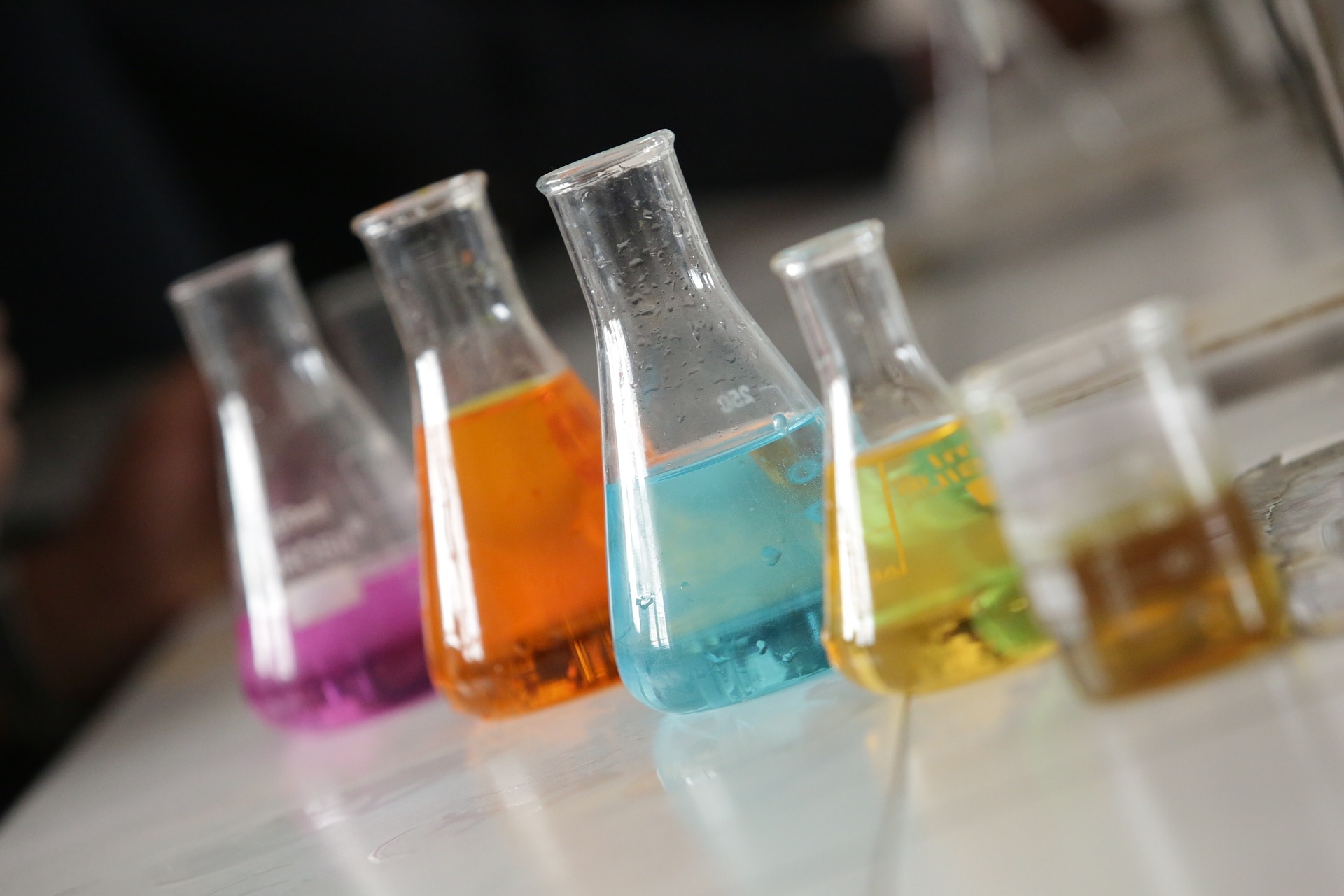# Physics Knowledge Can Be Helpful For Students Learning Chemistry

Title: Leveraging understanding of energy from physics to overcome unproductive intuitions in chemistry
Authors: Beth Lindsey, Megan Nagel, and Brandi Savani
First Author’s Institution: Pennsylvania State University
Status: Physical Review Physics Education Research – Open Access

Introduction

Chemistry is all about molecules attracting and repelling each other as they look for a low energy state. Without understanding how microscopic attractions lower the potential energy of a system, students can’t understand how atoms come together to form bonds, how positive charges and negative charges attract each other, or how solids become attracted to a solvent and dissolve.

Despite its importance, the concept of potential energy on a microscopic scale is quite abstract, and studies have shown that students in both introductory chemistry and physics classes struggle to understand it. Introductory physics classes often teach students about potential energy on a macroscopic scale, so students who have taken these classes might be familiar with how a falling object loses potential energy or how pulling magnets apart requires they gain potential energy. Today’s paper examines whether reminding students in an introductory chemistry course of these physics concepts improves their performance on questions about potential energy in chemistry.

Background

This study was inspired by the dual-process theory of reasoning. The dual process of reasoning posits that we come to conclusions by a two-part process. The first part of fast and intuitive, and it provides the first answer that pops into your head. The second process, called the analytical process, is slower. It is supposed to check the first process to see if the initial answer makes sense. The analytical process tends to be biased towards agreeing to the first fast “heuristic process,” however, so students often stick with an initial wrong answer unless there is a reason for the analytical process to veto it.

This research attempted to activate the analytical process to correct initial, incorrect, reasoning in a three-step process. First, students were shown a question about potential energy between two ions. Then, they were shown a new question that asked them to draw on their previous knowledge about potential energy in physics. Finally, the original ion question was presented again to see if the students had changed their original, often incorrect, presumptions.

Methods

The researchers probed students’ understanding of potential energy at microscopic scale with an “ions-question,” shown in figure 1. The correct answer was that the ions in Figure B have more potential energy. Once students provided there answer a text-box was provided for them to explain their reasoning.

Once students completed the first question, it was locked by the online quiz system and each student was randomly assigned one of two questions to do next. Half of students were assigned the question in Figure 2, which asked them to recall their understanding of gravitational potential energy from physics (The correct answer here was A).

Students who were not shown the book question were shown a question asking about the potential energy in magnets, which is shown in Figure 3. Researchers noted that while the wording of the magnet-question resembles the initial ions-question less than the book-question, students may have more of an intuitive sense of the relationship between magnets and ions than of the relationship between books in a gravitational field and ions. The correct answer was the potential energy increased as the magnets were moved apart. Once students answered and gave their answers to the second question, they were given the initial ions-question again to see if their answer changed.

Results

Out of 850 students who took the survey, only about 33% correctly answered the initial ions-question. Most students who answered incorrectly generally felt the ions had more potential energy when close together reasoned that the equation for energy between two charges has (R) in the denominator. As the charges were moved closer together, R would decrease and the potential energy would increase. They failed to understand the potential energy is negative for two ions of different charge, so the potential energy was actually becoming less negative as charges were moved farther apart.

78% of students who were given the second question about the potential energy of books answered it correctly, while only 36% of students given the magnet question answered it correctly. Students who were randomly assigned to the book question were more likely to answer the subsequent ion question correctly, with a 16% increase in students who answered the second ion-question correctly. Students who were shown the magnet question were actually slightly less likely to answer the second question correctly, although the decrease was not statistically significant. Even though students were more likely to answer the second ions-question correctly if they were given the books-question, they were less likely to recognize that the books question was similar to the ions-question than students given the magnets question were to recognize that the magnets and ions were similar. In both cases, students who recognized a similarity were more likely to answer the second ions-question correctly.

Takeaways

In this study, students understanding of how energy works on a microscopic scale was improved by a very minor intervention asking them to think about their knowledge of potential energy in their physics classes. The authors propose that the mechanism for this improvement acts through the analytic process as students are problem solving. Thinking about the potential energy of the book provided a check on the intuitive thought process that led students to erroneously conclude that ions which were closer together had higher potential energy. Since the intervention was so small, researchers are unsure of how long-lived of useful the improvements in students’ understanding of potential energy in chemistry will be.